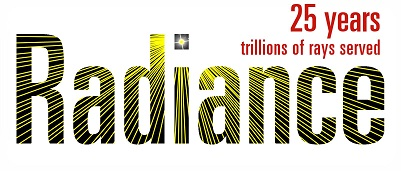# 5-phase method tutorial - section 6.1

Dear list,

Following example 1 in 5-phase method tutorial, I am trying to model
venetian blinds with frosted glass as blind material. The properties of the
glass are as follows:

Visible Transmittance: 67%
Reflectance: 18%
Clarity (small-angle scattering): 4%
Thickness: 8mm

To generate accurate BSDF files, I thought I should model the blind slats
as trans material, but I am struggling with translating above properties
into trans material modifiers.
I'd appreciate it if you helped me with writing the blind material script.
Also, I am not sure where to include the glass thickness. genblinds does
not seem to have an option for blind thickness.
Does the glass thickness in this case affect the BSDF results?

Thanks,
Leyla

Hi Leyla,

I was thinking someone else might answer this one, as a lot of folks have worked on similar things, but here goes...

Figuring out Radiance "trans" parameters is a classic challenge, and there are a few spreadsheets out there to make this a bit easier. The basic reference is section 5.2.6 from "Rendering with Radiance," attached below for your convenience. (Responders please delete attachment in your replies.)

Ts = .04
Td = .67 - .04 = .63
Rd = .18
Rs = 0 (guessing no clear reflections)

These yield:

A7 = Ts/(Td+Ts) = .04/(.63+.04) = .0597

A6 = (Td+Ts)/(Rd+Td+Ts) = (.63+.04)/(.18+.63+.04) = .7882

A5 = 0 (adjust if you want to scatter your transmitted rays a bit)

A4 = Rs = 0

A1 = A2 = A3 = .18/((1-0)*(1-A6) = .18/(1-.7882) = .85 (assumes uncolored material)

void trans frosted_glass
0
0
7 0.85 0.85 0.85 0 0 0.7882 0.0597

Regarding thickness, so long as the distance between slats is 16 cm or greater, you needn't worry about it. If they are closer together, you may want to use the xform -a option to create your own array of slats. It's actually pretty simple -- genblinds isn't doing that much for you with flat slats, anyway.

Cheers,
-Greg

.

TransParams.pdf (80 KB)

···

From: Leyla Sanati <leylasanati@gmail.com>
Date: August 29, 2013 10:00:45 PM PDT

Dear list,

Following example 1 in 5-phase method tutorial, I am trying to model venetian blinds with frosted glass as blind material. The properties of the glass are as follows:

Visible Transmittance: 67%
Reflectance: 18%
Clarity (small-angle scattering): 4%
Thickness: 8mm

To generate accurate BSDF files, I thought I should model the blind slats as trans material, but I am struggling with translating above properties into trans material modifiers.
I'd appreciate it if you helped me with writing the blind material script. Also, I am not sure where to include the glass thickness. genblinds does not seem to have an option for blind thickness.
Does the glass thickness in this case affect the BSDF results?

Thanks,
Leyla

1 Like

Thank you Greg. I really appreciate your help.

Leyla

···

On Fri, Aug 30, 2013 at 5:43 PM, Greg Ward <gregoryjward@gmail.com> wrote:

Hi Leyla,

I was thinking someone else might answer this one, as a lot of folks have
worked on similar things, but here goes...

Figuring out Radiance "trans" parameters is a classic challenge, and there
are a few spreadsheets out there to make this a bit easier. The basic
reference is section 5.2.6 from "Rendering with Radiance," attached below
replies.)

Ts = .04
Td = .67 - .04 = .63
Rd = .18
Rs = 0 (guessing no clear reflections)

These yield:

A7 = Ts/(Td+Ts) = .04/(.63+.04) = .0597

A6 = (Td+Ts)/(Rd+Td+Ts) = (.63+.04)/(.18+.63+.04) = .7882

A5 = 0 (adjust if you want to scatter your transmitted rays a bit)

A4 = Rs = 0

A1 = A2 = A3 = .18/((1-0)*(1-A6) = .18/(1-.7882) = .85 (assumes uncolored
material)

void trans frosted_glass
0
0
7 0.85 0.85 0.85 0 0 0.7882 0.0597

Regarding thickness, so long as the distance between slats is 16 cm or
greater, you needn't worry about it. If they are closer together, you may
want to use the xform -a option to create your own array of slats. It's
actually pretty simple -- genblinds isn't doing that much for you with flat
slats, anyway.

Cheers,
-Greg

.

*From: *Leyla Sanati <leylasanati@gmail.com>

*Date: *August 29, 2013 10:00:45 PM PDT

*
*

Dear list,

Following example 1 in 5-phase method tutorial, I am trying to model
venetian blinds with frosted glass as blind material. The properties of the
glass are as follows:

Visible Transmittance: 67%
Reflectance: 18%
Clarity (small-angle scattering): 4%
Thickness: 8mm

To generate accurate BSDF files, I thought I should model the blind slats
as trans material, but I am struggling with translating above properties
into trans material modifiers.
I'd appreciate it if you helped me with writing the blind material script.
Also, I am not sure where to include the glass thickness. genblinds does
not seem to have an option for blind thickness.
Does the glass thickness in this case affect the BSDF results?

Thanks,
Leyla

_______________________________________________